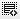## Pandas groupby count0

I am trying to get the proportion of one column. To do that I am using groupby() with count() i.e. similar to sql. But instead of getting one column count what i see is that i see count values in all columns.

here's how my data looks like:

``````import pandas as pd

df = pd.DataFrame({
'a': [1,2,3,4,5],
'b': ['a', 'a', 'b', 'c', 'd'],
'c': [False, True, True, False, True],
'd': [2006, 2006, 2007, 2008, 2006]
})

``````

When I do group by, i get count in each column:

``df.groupby('b').count()``
a c d
b
a 2 2 2
b 1 1 1
c 1 1 1
d 1 1 1

How do i get column count ?

Then i divide the result with length of data frame to get proportion and still get same number of columns

``df.groupby('b').count()/ len(df)``0

If I understand your question correctly, this should help:

``df.b.value_counts() / len(df)``

Remember: Count gives you number of non-null or non-empty rows in the column. This is not always equal to number of rows.0

Thank you GPS. This is what i was looking for. The values_counts() has same result as i was geting from group_by() + count() except not all columns.

What I am rying to solve is : "What proportion of rows occur in the column 'b' with the largest number of 'b'?"

Is the approach correct or do i need to apply some other formula?

Click on thisicon to add code snippet.